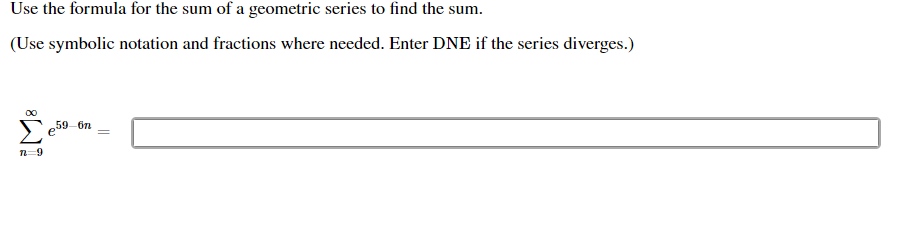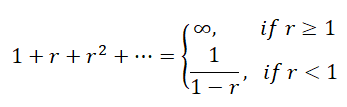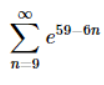# Use the formula for the sum of a geometric series to find the sum.(Use symbolic notation and fractions where needed. Enter DNE if the series diverges.)e59 onn=9

Question
1 viewshelp_outlineImage TranscriptioncloseUse the formula for the sum of a geometric series to find the sum. (Use symbolic notation and fractions where needed. Enter DNE if the series diverges.) e59 on n=9 fullscreen
check_circle

Step 1

We know that the sum of the geometric series is,.

Here, we have,.

...

### Want to see the full answer?

See Solution

#### Want to see this answer and more?

Solutions are written by subject experts who are available 24/7. Questions are typically answered within 1 hour.*

See Solution
*Response times may vary by subject and question.
Tagged in